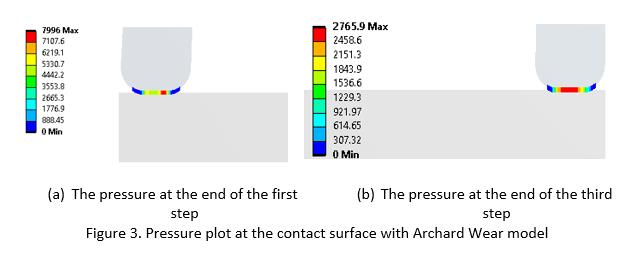## General Mechanical

•Ik93
Subscriber

Dear all,

I am trying to simulate the behavior of two cubes moving with respect to each other. The first cube is constrained in all directions while the second one is free to move along z-axis. Friction is applied at the interface between the two cubes and a pre-compression ensures that the two cubes remain in contact. If the pre-compression is too high, there is the chance of fracture (especially at one of the edges of the cubes). The problem is that I can't define in advance (i.e. using pre-meshed crack) where fracture will take place (if it does).

Is there any way to let Ansys identify whether fracture will occur and where?  If fracture does occur, it means that a part of the cube will fall apart hence the surface area where friction takes place is decreased. That is the aim of the simulation. Do you know if I can perform such a simulation using Ansys?

•Wenlong
Ansys Employee

Hi,

This can probably be simulated using contact wear in ANSYS. Contact wear can change the friction coefficient based on nodal stress, contact pressure and so on. A popular model used for contact wear is Archard Wear model. A user can define his own wear model as well. Contact wear can also change the geometry of the contact surface.

The image below illustrates the idea of contact wear: